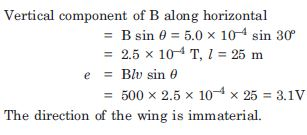# NCERT Solutions for Class 12 Physics Chapter 6 - Electromagnetic Induction

Students gearing up for their Class 12th board exam preparation can utilize NCERT Solutions to get started with learning. The solutions are effective in a way that they provide a thorough understanding of the entire chapter. The subject matter experts at Aasoka have made sure to use simple, easy, and easy-to-understand language for NCERT Solutions for Class 12th.

Physics chapter 6th “Electromagnetic Induction” discusses magnetism and electricity together. Lenz’s and Faraday’s laws are the main concepts of this chapter. Students will be able to clearly understand the topics of the chapter with the help of problems and examples based on the concept.

##### Question 1:

Predict the direction of induced current in the situations described by the following Fig. TBQ 6.1 a to f :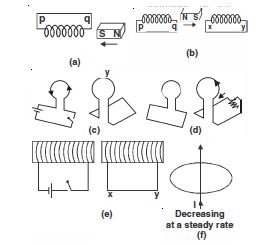According to Lenz’s law south pole develops at q, current induced must be clockwise at q.

$\therefore$ In the coil, induced current is from p to q.

Coil pq in this case would develop S-pole at q and coil xy would also develop S pole at x. Therefore, induced current in the coil pq will be from q to p and induced current in coil xy will be from y to x.

Induced current in the right loop will be along xyz.

Induced current in the left loop will be along xyz.

Induced current in the right coil is from x to y. No induced current since fixed lines lie in the plane of the loop.

##### Question 2:

Use Lenz’s law to determine the direction of induced current in the situations described by the figures given below :

(a) a wire irregular shape turning into a circular shape.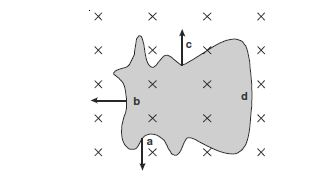a circular loop being deformed into a narrow straight wire. The cross (×) indicates magnetic field into the paper and the dot ($·$) indicates the magnetic field out of the paper.

Induced current is along adcba. As shape of loop changes from irregular to circular, its area increases. Flux through it increases. Induced current would flow so as to oppose the applied magnetic field and decrease the flux. It will produce outward flux.

Induced current is along adcba. As shape of loop changes from circular to a narrow straight wire, its area decreases. Flux through it decreases.

Induced current would flow so as to favour the applied magnetic field and increase the flux. It will produce an outward flux (as being produced by outward action of applied magnetic field).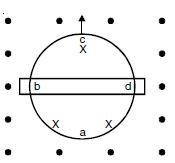##### Question 3:

A long solenoid with 15 turns per cm has a small loop of area 2.0 cm2 placed inside the solenoid normal to its axis. If the current carried by the solenoid changes steadily from 2.0 A to 4.0 A in 0.1 s, what is the induced voltage in the loop while the current is changing ?

Given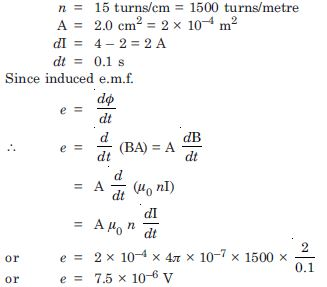##### Question 4:

A rectangular wire loop of sides 8 cm and 2 cm (Fig. 6.9) with a small cut is moving out of a region of uniform magnetic field of magnitude 0.3 T directed normal to the loop. What is the emf developed across the cut if the velocity of the loop is 1 cm s–1 in a direction normal to the :

longer side, shorter side of the loop ? For how long does the induced voltage last in each case ?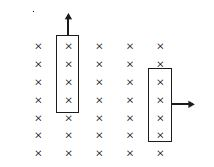B = 0.3 T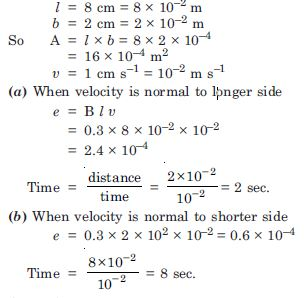##### Question 5:

A 1.0 m long metallic rod rotates with an angular frequency of 400 rad s–1 about an axis normal to the rod passing through its one end. The other end of the rod is in contact with a circular metallic ring. A constant magnetic field of 0.5 T parallel to the axis exists everywhere. Calculate the emf developed between the centre and the ring.

Given l = 1m, $\mathrm{\omega }$ = 400 s–1
B = 0.5 T, e = ?
Here one end of the rod has zero velocity and the other end has velocity (l$\mathrm{\omega }$)
∴ Average velocity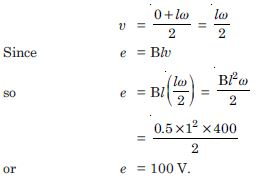##### Question 6:

A circular coil of radius 8.0 cm and 20 turns rotates about its vertical diameter with an angular speed of 50 rad s–1 in a uniform horizontal magnetic field of magnitude 3.0 × 10–2 T. Obtain the maximum and the average e.m.f. induced in the coil. If the coil forms a closed loop of resistance 10 $\mathrm{\Omega }$, calculate the maximum value of current in the coil. Calculate the average power loss due to Joule heating. Where does this power come from ?

Flux through each turn of the loop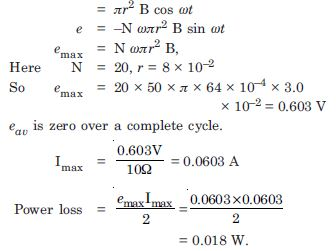The induced current causes a torque opposing the rotation of the coil. An external agent (a rotor) must supply torque (and do work) to counter this torque in order to keep the coil rotating uniformly. Thus the source of the power dissipated as heat in the coil is the external rotor.

##### Question 7:

A horizontal straight wire 10 m long extending from east to west is falling with a speed of 5.0 ms–1 at right angle to the horizontal component of the earth’s magnetic field 0.3 × 10–4 Wb m2.

What is the instantaneous value of the e.m.f. induced in the wire ?
What is the direction of the e.m.f. ?
(c) Which end of the wire is at the higher electrical potential ?

l = 10 m, $\mathrm{\upsilon }$ = 5.0 ms–1,
B = 0.30 × 10–4 Wb m2
Instantaneous value of e.m.f. induced e = Bl$\mathrm{\upsilon }$ = 0.30 × 10–4 × 10 × 5.0
or e = 1.5 × 10–3 V
The direction of e.m.f. is from east to west. Since the current is flowing from east to west so the eastern end is at higher potential.

##### Question 8:

Current in a circuit falls from 5.0 A to 0.0 A in 0.1s. If an average e.m.f. of 200 V induced, give an estimate of the self inductance of the circuit.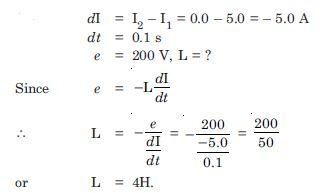##### Question 9:

A pair of adjacent coils has a mutual inductance of 1.5 H. If the current in one coil changes from 0 to 20A in 0.5 s, what is the change of flux linkage with the other coil ?

M = 1.5 H,
dI = I2– I1
= 20 – 0 = 20 A
dt = 0.5s, d$\mathrm{\phi }$ = ?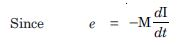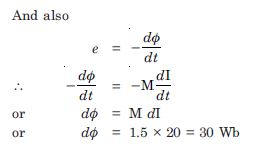##### Question 10:

A jet plane is travelling towards west at a speed of 1800 km h–1. What is the voltage difference developed between the ends of the wing 25 m long, if the earth’s magnetic field at the location has a magnitude of 5.0 × 10–4 and the dip angle is 30$°$ ?

$\mathrm{\upsilon }$ = 1800 km h–1 = 500 ms–1